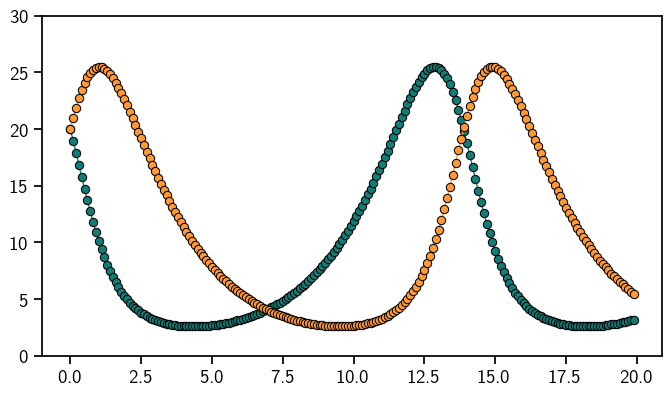# Tutorials¶

Learn how to use ProbNum and get to know its features. You can interactively try out thedirectly in the browser or by downloading the notebooks from the GitHub repository.

## Getting Started¶## Features of ProbNum¶

### Probability¶

Random variables are the main objects in ProbNum. They represent uncertainty about a numerical quantity via their distribution. A probabilistic numerical method takes random variables as inputs and also outputs random variables.### Linear Algebra¶

Linear algebra is fundamental to arguably most of scientific computation. ProbNum defines (finite-dimensional) linear operators in a memory-efficient manner and implements probabilistic numerical methods to solve linear systems.### Ordinary Differential Equations¶

The behaviour of complex systems which evolve over time is often described via the use of ordinary differential equations. ProbNum provides a set of methods to solve ordinary differential equations based on filtering approaches which quantify the uncertainty introduced by discretization.### Bayesian Filtering and Smoothing¶

Bayesian filtering and smoothing provides a framework for efficient inference in state space models. For non-linear state space components, ProbNum provides linearization techniques that enables Gaussian filtering and smoothing in more complex dynamical systems.